# A new formula to compute the n'th binary digit of pi

Fabrice Bellard

January 20, 1997

We describe here a mean to find formulas similar to those in . We show in particular that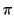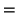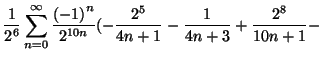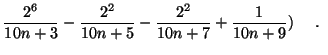(1)

This formula is very interesting because, with the algorithm described in , it enables us to compute the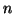th binary digit of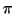43% faster than the previous known formula :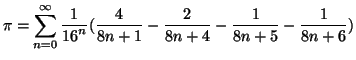(2)

The method to get formulas such as (1) is in fact very simple. We use that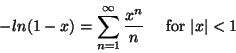and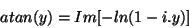for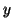real.

In particular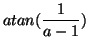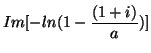(3)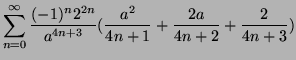and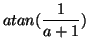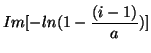(4)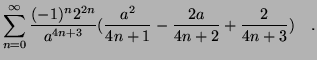With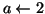in (3) we get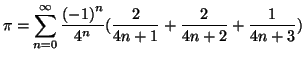(5)

which is mentionned in .

Some classical arctangent relations give interesting results: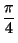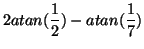(6)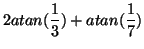(7)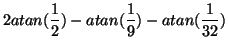(8)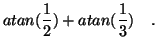(9)

In particular, we obtain from (6) and (3)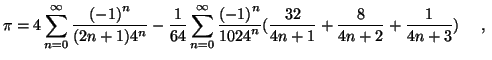which gives (1) by reordering the terms.

The existence of a formula faster than (1) to calculate theth binary digit ofremains an open question.

## Bibliography

1
David H. Bailey, Peter B. Borwein and Simon Plouffe, On the Rapid Computation of Various Polylogarithmic Constants, to appear in April 1997 in Mathematics of Computation.

Thu Feb 15 23:31:20 CET 2007
Fabrice Bellard (http://bellard.org)